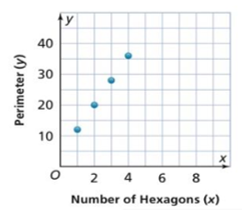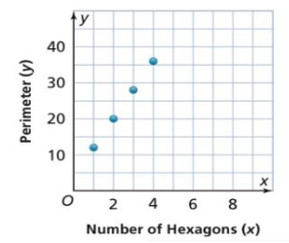Maths-
General
Easy

Question

# The relationship between the number of hexagons, , and the perimeter of the figure they form, , shown in the graph. Is the perimeter of the figure a function of the number of hexagons? Explain.Hint:

## The correct answer is: graph is a function.

### Step by step solution:The given graph isWe join the points by a smooth curve in the above graph.We can check if a graph is a function by the vertical line test. A graph represents a function if any vertical line in the xy plane cuts the graph at maximum one point. We use this method to check if the given graph represents a function.We can see that if we draw any vertical line in the xy plane, it cuts the graph at exactly one point.Hence, the given graph is a function.

In a function, every value of x must have one value of y. When we draw a vertical, it cuts x axis at one point, we take that point to be x. If the vertical line cuts the graph at two points, then it gives two values of y for one value of x. This does not represent a function.#### With Turito Foundation.#### Get an Expert Advice From Turito.